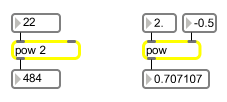# pow

Computes x to the power of y

## Description

pow raises the base value (set in the left inlet) to the power of the exponent (set in the right inlet).

## Arguments

Name Type Opt Description
base-value float or int opt Sets the base value. The default value is 0.

## Messages

 bang Causes pow to output the most recent value it has calculated. int base/exponent-value [int] In left inlet: Sets the exponent. In right inlet: Sets the base value. float base/exponent-value [float] In left inlet: Sets the exponent. In right inlet: Sets the base value. (inlet1) base-value [float] In right inlet: Sets the exponent. list exponent and base values [list] A list consisting of the base-value followed by the exponent-value will cause pow to output the base-value raised to the power of the exponent.

## Output

float: The base value (from the left inlet) raised to the exponent (from the right inlet).

## Examplespow will give you a square deal (and other numbers too)